Ru

# Quil Intro

This is an introductory post about Quil. Quil is an interactive animation library for clojure. Simply speaking it allows you to draw whatever you want on a rectangular window. Quil provides tons of useful functions for drawing in 2D and 3D. In this post I will show how to create and run Quil sketches. Let's start with something simple: with trigonometry... Everyone loves trigonometry: sine, cosine, tangent, what can be better? Our first sketch will draw a spiral using sin and cos functions:

project.clj

``````(defproject quil-intro "0.1.0-SNAPSHOT"
:dependencies [[org.clojure/clojure "1.8.0"]
[quil "2.5.0"]])
``````

quil_intro.clj:

``````(ns quil-intro
(:require [quil.core :as q]))

; define function which draws spiral
(defn draw []
; make background white
(q/background 255)

; move origin point to centre of the sketch
; by default origin is in the left top corner
(q/with-translation [(/ (q/width) 2) (/ (q/height) 2)]
; parameter t goes 0, 0.01, 0.02, ..., 99.99, 100
(doseq [t (range 0 100 0.01)]
; draw a point with x = t * sin(t) and y = t * cos(t)
(q/point (* t (q/sin t))
(* t (q/cos t))))))

; run sketch
(q/defsketch trigonometry
:size [300 300]
:draw draw)
``````

As you can see for basic sketch we need to define a `draw` function which, well, draws something. Then we call `defsketch` passing `draw` function to it. Here is the image drawn by our code:Beautiful, isn't it?

Now let's refactor `draw` function making plotting functions easier. To achieve this we'll define `draw-plot` function which takes a parametric function f(t) = (x, y) , ranges for parameter t and plots given function over given range. Here is refactored code:

``````; define f
(defn f [t]
[(* t (q/sin t))
(* t (q/cos t))])

(defn draw-plot [f from to step]
(doseq [two-points (->> (range from to step)
(map f)
(partition 2 1))]
; we could use 'point' function to draw a point
; but let's rather draw a line which connects 2 points of the plot
(apply q/line two-points)))

(defn draw []
(q/background 255)
(q/with-translation [(/ (q/width) 2) (/ (q/height) 2)]
(draw-plot f 0 100 0.01)))
``````

Cool, now we can experiment with `f` by changing it in any way we'd like. And here comes real beauty of Quil: live reloading.

After we changed code we don't need to close sketch, recompile everything and start sketch again as we would do in most other languages. In quil we can update all functions on the fly and see results immediately. In fact we can program whole sketch from the beginning to the end without ever closing it. Of course there are some things we can't do on the fly. We can't register mouse and keyboard listeners on the fly, but we still can update already registered. Now let's get back to work and update `f` function:

``````; you can get awesome plots using random combinations of trigonometric functions
; here f which plots a flower
(defn f [t]
(let [r (* 200 (q/sin t) (q/cos t))]
[(* r (q/sin (* t 0.2)))
(* r (q/cos (* t 0.2)))]))
``````

Now we need to reload updated `f`. Standard clojure techniques can be used to do it:

• Emacs: `C-x C-e` to reload `f`.
• LightTable: `Ctrl+Enter` to reload `f`.
• REPL: redefine `f` function.

And here is the flower (together with some other plots of random functions):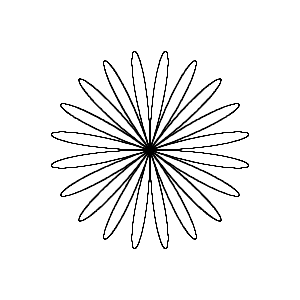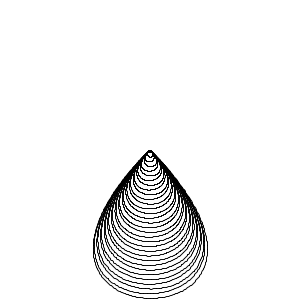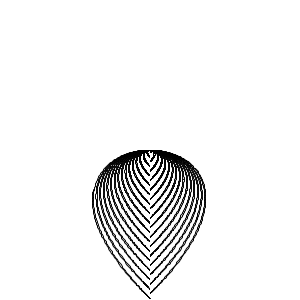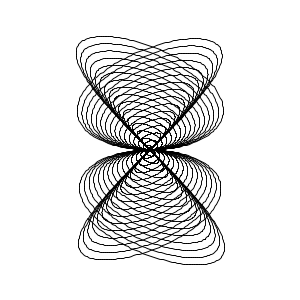### Animation

Now we'll look at another quil feature. Up to this point we drew static images which weren't changing over time. But in fact `draw` function is called repeatedly in short intervals. That means we can draw moving objects and do real animation! Let's modify our sketch so on each iteration only a part of a plot is drawn: line from f(t) to f(t+1). The only problem is that t should change on each iteration, to solve it we'll use `frame-count` which returns current iteration number and we can use this number as t. Here is implementation:

``````(defn draw []
(q/with-translation [(/ (q/width) 2) (/ (q/height) 2)]
; note that we don't use draw-plot here as we need
; to draw only small part of a plot on each iteration
(let [t (/ (q/frame-count) 10)]
(q/line (f t)
(f (+ t 0.1))))))

; 'setup' is a cousin of 'draw' function
; setup initialises sketch and it is called only once
; before draw called for the first time
(defn setup []
; draw will be called 60 times per second
(q/frame-rate 60)
; set background to white colour only in the setup
; otherwise each invocation of 'draw' would clear sketch completely
(q/background 255))

(q/defsketch trigonometry
:size [300 300]
:setup setup
:draw draw)
``````

Time for animation!All our sketches are black and white. It would be nice to add some colours. I'll leave it as exercise to reader, or, if you're too lazy, you can simply check GitHub repo in the end of this post. Here is what I came up with: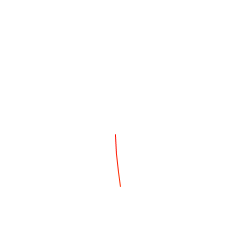That's it for today. Some final notes: Quil is based on Processing programming language, which is itself wonderful language/program for creating visual arts, but Quil moves it to the next level with live reloading (the same could be said about general programming and clojure). It is very cool to be able to reload parts of your sketch on fly and get immediate feedback. It boosts your experimentation velocity so I would definitely encourage everyone to play with it. Here is some useful links related to this post: